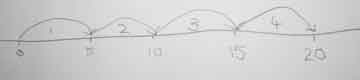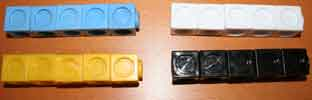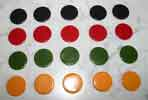#### You may also like### I'm Eight

Find a great variety of ways of asking questions which make 8.### Let's Investigate Triangles

Vincent and Tara are making triangles with the class construction set. They have a pile of strips of different lengths. How many different triangles can they make?### Noah

Noah saw 12 legs walk by into the Ark. How many creatures did he see?

# Odd Times Even

## Odd Times Even

Choose any two numbers, such as 4 and 5. One must be even and the other odd.

Try multiplying them together. How could you show this?

Lewis used a number line:Athol used counters:Look closely at one of these models.

Can you see anything in it that would work in exactly the same way if you used the same model  with a different pair of even and odd numbers?

Can you use your one example to prove what will happen every time you multiply an even number and an odd number together?

See if you can explain this to someone else.

Are they convinced by your argument?

Once you can convince someone else, see if you can find a way to show us your argument. You might draw something or take a photo of things you have used to prove that your result is always true from your example.

### Why do this problem?

This problem supports the development of the idea of generic proof with the children. This is a tricky concept to grasp but it draws attention to mathematical structures that are not often addressed at primary school level. In essence it explores one example to unpack the underlying structure in the mathematics that proves the general result. It is possible that only very few children in the class may grasp the idea but this is still a worthwhile activity which provides opportunities for children to explore odd and even numbers and the relationship between them. Proof is a fundamental idea in mathematics and in helping them to start this problem you will be helping them to behave like mathematicians.

By addressing the case of multiplying an even and odd number, a generic proof that multiplying an even and an odd number gives an even answer is developed based on the structure of odd and even numbers. The article entitled Take One Example will help you understand how this problem supports the development of the idea of generic proof with the children. Reading it will help you to see what is involved.

### Possible approach

Invite the children to work in pairs. Ask each pair to choose an even and an odd number and multiply them together. It is important that the children are free to choose their own numbers, but it is probably most helpful if they choose numbers that are easy to model and that they are secure with. That is why we suggest the numbers 4 and 5 as our example in the question.

Suggest that they make a model of their numbers using apparatus that is widely available in the classroom. Resist pointing them to a particular type of apparatus or  specific kind of diagram, unless they become stuck. It is important that they explore which apparatus is helpful, and which is not, as part of looking for the general structure in the particular example they have chosen. Encourage different pairs to share how useful (or not) they are finding a particular piece of apparatus. Resources such as Multilink cubes, squared paper or Numicon will turn out to be helpful. Some children may spend some time exploring creating models of their calculation and that may be valuable for them in developing their understandng of multiplication. Others may choose a model easily and move on to considering the structure of the example more quickly.

The challenge for the children is to see if they can see the general structure within their one chosen example and explain what they see in a convincing way.

### Key questions

How would you like to represent these numbers being multiplied together?
Can you see anything in your example that would work in exactly the same way if you used a different pair of odd and even numbers?
Can you say what will happen every time you multiply an even number by an odd number?
Can you convince your friend that this is true?

### Possible extension

What will happen if you multiply an even number by an odd number?

Can you prove what will happen in a similar way to when you multiplied an odd number by an even number? Does the order matter?

What will happen if you multiply an even number by an even number? Will the oddness or evenness of the result always be the same? Can you prove it?

How about multiplying an odd number by and odd number? Can you find the general result here? Can you prove it by examining one example?

### Possible support

It may be helpful to encourage children to use squared paper and place counters in the squares to represent their calculation. This may help them to see the structure more clearly and the connection of the timesing to repeated addition. You will be able to look at the array one way or the other and see that it is the sum of  a number of even numbers and therefore must be even.